There is a lot that you can do with a spreadsheet beyond simple sums and averages. If you are in business or science, you learn these more complicated functions quickly because everyone around you knows about them and uses them, but if you are in another field, they will probably remain a mystery to you.

My goal is to write a series of short tutorials about some of the nifty things you can do with spreadsheets. A lot of this information will be about using formulas, but I will cover other topics as well. I’m assuming that you know how to open a spreadsheet, enter some data, and print it out, and that you know what cells, columns, and rows are. You should also know that I’m using OpenOffice for these demos and screen caps. Most of these tips and techniques should work the same in Microsoft Excel.

I recently upgraded to a new laptop, giving me the opportunity to combine the iTunes libraries from two different computers into one. When I was finished, I saw this at the bottom of my iTunes window: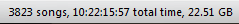Interesting, I thought. That translates to 10 days, 22 hours, 15 minutes, and 57 seconds of music. My music collection is a mixture of pop, rock, jazz, classical, and podcasts, so I started wondering what the average song length was. As it turns out, that was fairly easy to calculate.

## Calculating the Total Time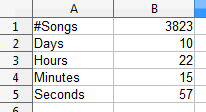To calculate an average, we need to know the total time in seconds and work backward from there. I used column C to calculate seconds. To get a spreadsheet to calculate things, we enter an equal sign (=) first. I didn’t need to do anything to the seconds, so I entered an equal sign in C5 and then clicked on cell B5: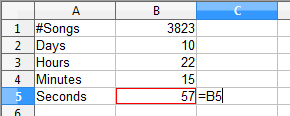The red box around B5 shows that I clicked in it. The formula in C5 basically says “Take whatever value is in B5 and put it here”. Hitting the enter key gave me this:To calculate the minutes in seconds, we need to do a bit of multiplication. In C4, entered an equal sign, and then clicked on B4, just like I did with seconds: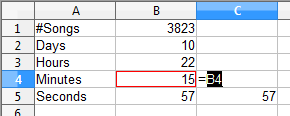I then typed an asterisk (which is what computers use to multiply; it doesn’t matter whether you use Shift+8 or the numeric keypad) followed by the number 60: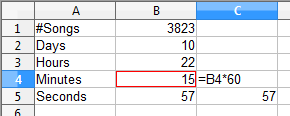What this says is “Take the value from B4, multiply it by 60, and put that value in C4”. After hitting the enter key, things looked like this: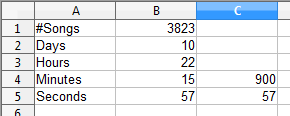For hours, we need to multiply by 3600, which is sixty seconds per minute and sixty minutes per hour. However, we can also just let the spreadsheet do this multiplication for us:And we can do something similar for the days, which is 60*60*24: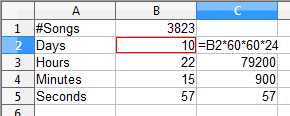Now we need to total all those seconds. There are two ways to do this.

The first is to select the cell where we want our total and use the SUM formula, which adds things up. I went to C7, entered an equal sign (remember that we always start with an equal sign if we want the spreadsheet to do calculations), typed the word SUM and an opening parenthesis: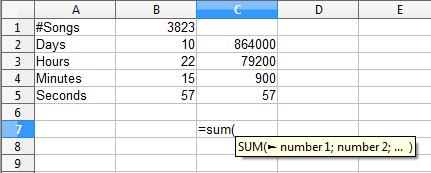OpenOffice is nice, because as soon as I did that, it showed me that little pop-up explaining the SUM function:This tells me two things. First, that I typed the formula correctly, and second, the actual format for the SUM function. In other words, whatever I want to sum, I can just enclose between parentheses, separating each value with a semicolon. Again, here we have options.

### SUM Method #1

From this point, I can just click on the first cell I want to add (C2), type a semicolon, click on the second cell (C3), type a semicolon, and so on. The result looks like this:Hitting the enter key gives us this: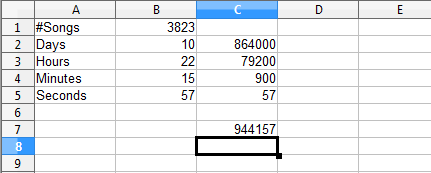Somehow I thought it would be more seconds than that.

### SUM Method #2

As useful as that is, it gets a bit tedious if there are more than a few cells to be added. It’s much quicker to use this method: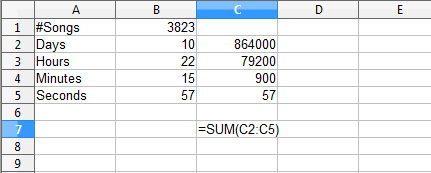Since all of my data is in a single column (and when working with a spreadsheet, your data is usually in a single column or row), I can just click on the first cell in the series (C2), type a colon (not a semicolon), and then click on the last cell in the series, which in this case is C5. Typing a closing parenthesis and hitting the enter key gives us the same result:I can achieve the same thing without even typing. After typing `=SUM(`, I can click on the first cell, and while holding the mouse button down, drag down the column to the last cell, where I then release the mouse button. It looks a bit like this: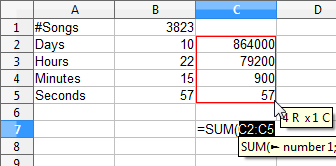We get the same result either way.

## Calculating the Average Time

To calculate an average, we simply divide the total time by the number of songs. In B8, I typed “Average” and in C8, I entered the formula to calculate the average: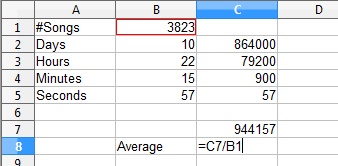I started by entering an equal sign, which tells the spreadsheet that we want it to calculate something, then clicking in C7 (which contains the total time), typing a division symbol (/), and finally clicking in the cell with the total number of songs (B2). Hitting the enter key gives us our average: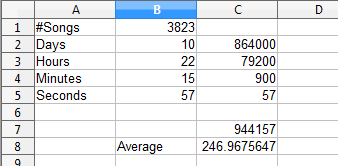which is 246.9675647 seconds per song.

## Calculating a Useful Average

Unfortunately, that number really doesn’t tell us much. We need to do something with that data to get it into a form that means something to the average human being. That will be the subject of our next tutorial, which is here.